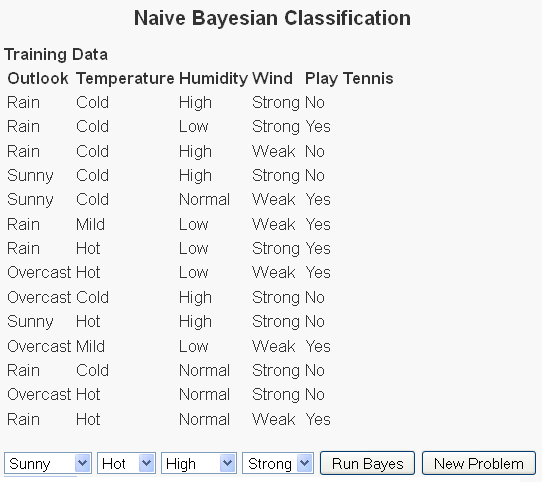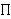# Naive Bayesian ClassificationSuppose there is a sequence of events that took place e1, …, ene, with each event belonging to a certain classification group g1, …, gng. Then the problem of determining which of these groups a new event belongs is the classification problem.

A naive Bayes classifier will determine to which of the possible classification groups a new observation belongs with the (naive) assumption that every feature of this new observation has no relationship to any other feature of this observation.

This assumption of independence of the columns of the feature vector allows us to use Bayes’ Theorem to determine the probability that the new observation will belong to each of the observation groups. Bayes’ Theorem will then say that the probability this new observation belongs to a classification group, given the features is equal to the probability of the occurrence of that classification group in the observed data (i.e. P(C)) multiplied by the conditional probability of the joint distribution of the features given the same classification group P(F1, …, Fnf. The naive assumption allows us to quickly calculate the joint distribution of the features, given the classification group as the product of each feature given that same classification group.

This can be written as:

P(C | F1, …, Ffn) =
 P(C) P(F1, …, Ffn | C) P(F1, …, Ffn)
= P(C) P(F1 | C) * … * P(Ffn | C
 P(C) P(F1, …, Ffn | C) P(F1, …, Ffn)
= P(C)i = 1 to nfP(Fi | C)
 P(C) P(F1, …, Ffn | C) P(F1, …, Ffn)

So suppose we have observations that give the following data:
P(F1 | N) = 0.286
P(F2 | N) = 0.143
P(F3 | N) = 0.429
P(F4 | N) = 0.429

P(F1 | Y) = 0.143
P(F2 | Y) = 0.571
P(F3 | Y) = 0.571
P(F4 | Y) = 0.571

P(Y) = 0.5
P(N) = 0.5

Then P(N | F1, F2, F3, F4) = (0.5 * 0.286 * 0.143 * 0.429 * 0.429)
= 0.008

Then P(Y | F1, F2, F3, F4) = (0.5 * 0.143 * 0.571 * 0.571 * 0.571)
= 0.001

After we normalize the two terms, we wind up with Then P(N | F1, F2, F3, F4) = .2204

and

Then P(Y | F1, F2, F3, F4) = .7796

So the naive Bayes classifier says that the likely classification group for this observation is Y.

Check out my examples page for more examples of the naive Bayes classifier.